# Why Expiration and Strike Prices Are Important

Vertical credit spreads are often sold out-of-the-money (OTM). In other words, the trader thinks the stock will stay above or below a certain area. For example, with the stock at \$85, the trader believes the stock will at least trade above \$80. The trader can sell an 80 put and buy a 75 put. The trade benefits if the stock moves higher or time passes (theta). Theta measures the decline of an option’s value through the passing of time. Max profit is earned if the stock closes at \$80 or higher at expiration. In effect, the stock has three out of four ways to profit: a move higher, trading sideways or even a move lower.

Synthetically, vertical debit and credit spreads are the same. Take a look at the option chain below.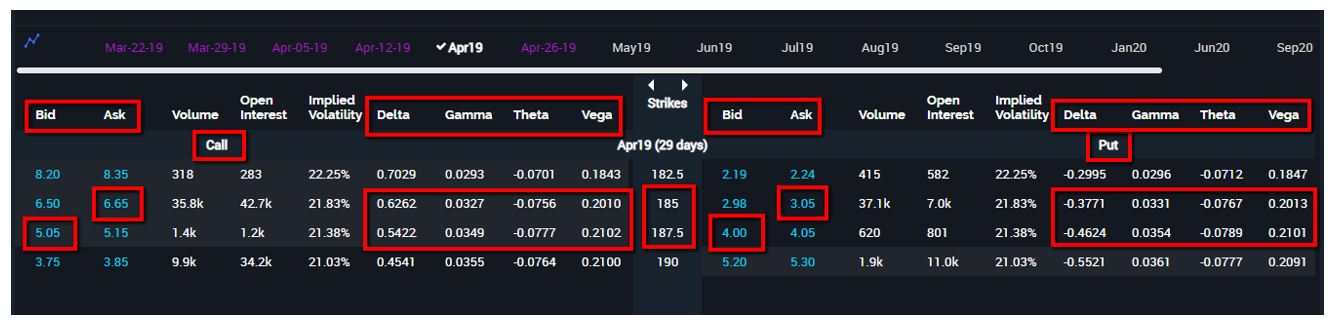The 185/187.5 vertical call debit spread (bull call) is essentially exactly the same as the 185/187.5 vertical put credit spread (bull put). The max profit on the bull call is 0.90 (2.50 (diff in strikes) – 1.60 (cost of the spread)). The max profit on the bull put is 0.95 (4 – 3.05) from the credit received. The slight discrepancy could be due to the bid/ask spreads. Even the greeks are very similar and synthetically the same too. This is exactly why option traders who trade vertical debit spreads need to start thinking in this manner. Theta can be just as important for profit as it is for vertical credit spreads.

Before going further, let’s define the other option greeks besides theta. Delta changes the premium based on the underlying. Keeping it simple, for every dollar change in the underlying, delta will change the premium by its amount. Gamma changes the delta based on the underlying. Keeping it simple once more, for every dollar change in the underlying, gamma will change delta by its amount. And to finish off the four major option greeks, there is vega. Vega measure the option’s rate of sensitivity to volatility. Keeping it simple yet again, for every 1% change in implied volatility, the premium will change by that amount. You are now almost an option greek expert, so let’s continue.

When an option trader buys a vertical debit spread, there will be positive and negative greeks. Take, for example, a bull call spread (vertical call debit). A long call is purchased and a higher strike call is sold with the same expiration. There is positive delta from the long call and negative delta from the short higher strike call. There is positive gamma from the long call and negative gamma from the short call. There is negative theta from the long call and positive theta from the short call. Lastly, there is positive vega from the long call and negative vega from the short call. Whatever greek is larger (between the positive and negative) controls the trade. For a vertical debit spread, positive will not change during the life of the trade. If it is a bullish trade (bull call), delta will always stay positive, and if it is a bearish trade (bear put), delta will remain negative.

Gamma, theta and vega may change during the course of the trade depending on the underlying and time. This is where an option trader can gain an edge particularly when it comes to theta. Theta can either start out as positive or move from negative to positive throughout the trade. When theta is positive, time passing helps the position to profit for either debit or credit spreads. This will be explained in greater detail as an example is examined.

Positive theta can be just as important for vertical debit spreads as it is for vertical credit spreads. It is understandable that most option traders focus on the positive theta when it comes to OTM credit spreads. Theta is positive or becomes positive for vertical debit spreads when the underlying is past the breakeven point of the trade. Think about it, if the stock is past breakeven, only positive theta will keep the spread from losing money.

For example, if a 45/50 vertical call spread (bull call) was purchased for 2.50, which means the breakeven is \$47.50 (45 + 2.50), and the underlying is currently at \$47.75 and closes there at expiration, delta would not have been a factor because the stock stayed the same from the purchase to expiration. Positive theta would have produced a 0.25 (47.75 – 47.50) profit because of the intrinsic value of the long call. If the spread starts off with positive theta, it will only turn negative if the underlying moves back below the breakeven point of the trade. Positive theta can be just as and sometimes even more important for vertical debit spreads if delta does not do most of the profit heavy lifting.

Let’s take a look at an example where a bear put spread (vertical debit) could be used when an option trader is expecting a move lower. If the trader is expecting a move lower sooner than later, a shorter expiration might be a smart choice. With the stock at around \$166.36, an option trader could choose an expiration with 7 days to go or even longer. For this example, an expiration with 14 days to go was chosen to compare the two. This example is meant to show how one week could make a difference. Take a look at the option chain below.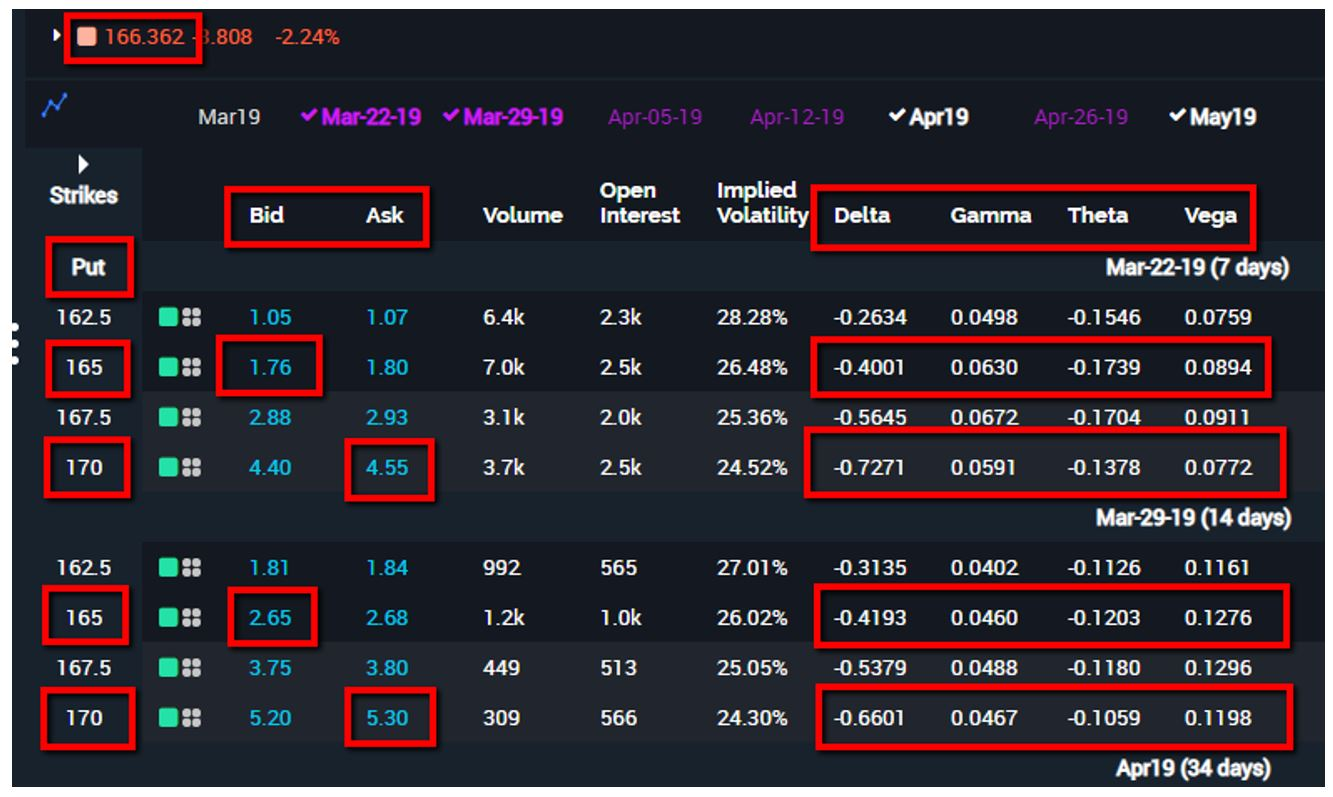The 165/170 bear put spread was chosen for each with 7 and 14 days to go until expiration. The 170 put is bought and the 165 put is sold. Maximum profit is earned at \$165 or lower for each and maximum loss is realized at \$170 or higher at expiration for each.

The Mar-22 (7 days) expiration has a cost and maximum risk of 2.79 (4.55 – 1.76), which means the maximum profit is 2.21 (5 – 2.79). The Mar-29 (14 days) expiration has a cost and maximum risk of 2.65 (5.30 – 2.65), which means the maximum profit is 2.35 (5 – 2.65). Take a look at the delta above. The shorter expiration has a delta close to -0.33 (0.4001 – 0.7271) versus -0.24 (0.4193 – 0.6601) for the longer. The stock will realize a bigger gain based on delta with the shorter expiration. The reason is that delta gravitates toward 0.50 the further out to expiration. The longer the expiration, the smaller the delta difference is between the strikes.

But that is not the only option greek with a discrepancy. Theta also plays a key role. Since the spread is in-the-money (ITM) and below the breakeven point for each spread, the position already has positive theta. The shorter expiration has a positive theta close to 0.03 (0.1739 – 0.1378) versus 0.01 (0.1203 – 0.1059) for the longer. Not a huge discrepancy for either delta or theta but this will change especially if the underlying falls and time passes. As good fortune would have it, the stock drops down to \$160.47 several days later. Take a look at the option chain after the drop and time has passed below.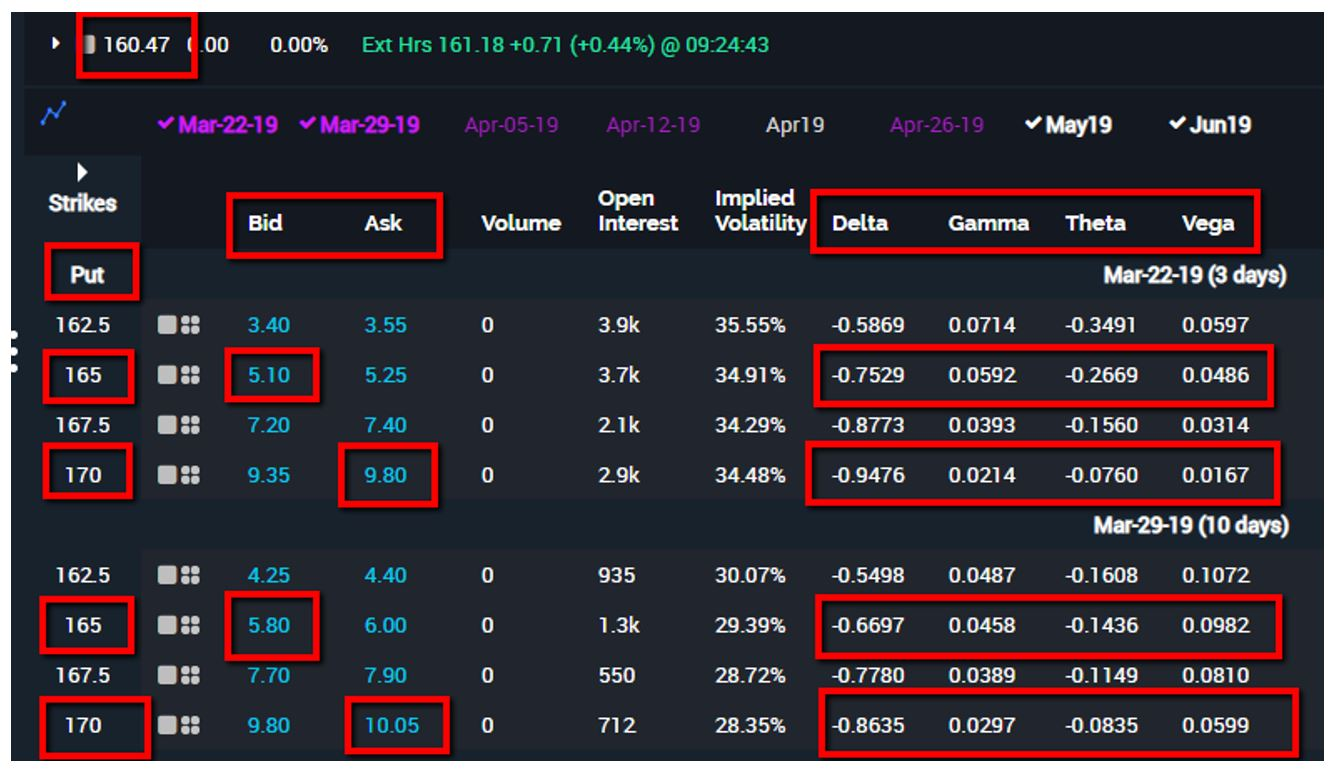A profit of 1.91 (4.70 – 2.79) or \$191 in real terms is realized on the Mar-22 expiration spread based on the ask price of the spread. A profit of 1.60 (4.25 – 2.65) or \$160 in real terms is realized on the Mar-29 expiration spread based on the ask price of the spread. Although as the underlying moved lower the delta became similar for each expiration, look what happened to theta. They both increased to an even more positive theta but the shorter expiration is currently around 0.19 (0.2669 – 0.0760) versus around 0.06 (0.1436 – 0.0835) for the longer expiration. That is a positive theta difference close to 0.13 (0.19 – 0.13). That might not seem like much but if 10 contracts were purchased, positive theta contributed an extra \$130 (0.13 X 100) to the profit, which can be directly attributed to choosing a shorter expiration that matches the expected move. Now that is exciting!

As noted above, choosing strikes can be vitally important for vertical debit spreads too. Many times, as in the previous example above, moving the spread deeper ITM makes sense despite a higher risk and lower reward trade. Let’s examine another scenario where there is an expected move higher in a short amount of time.

A stock is currently trading at \$187.18 and is forecast to move higher in a short amount of time. An expiration of 7 days is chosen and the options have 2.50 strike increments to choose from. An option trader is considering either a 185/187.5 or 187.5/190 bull call spread (vertical call debit). Take a look at the first option chain below.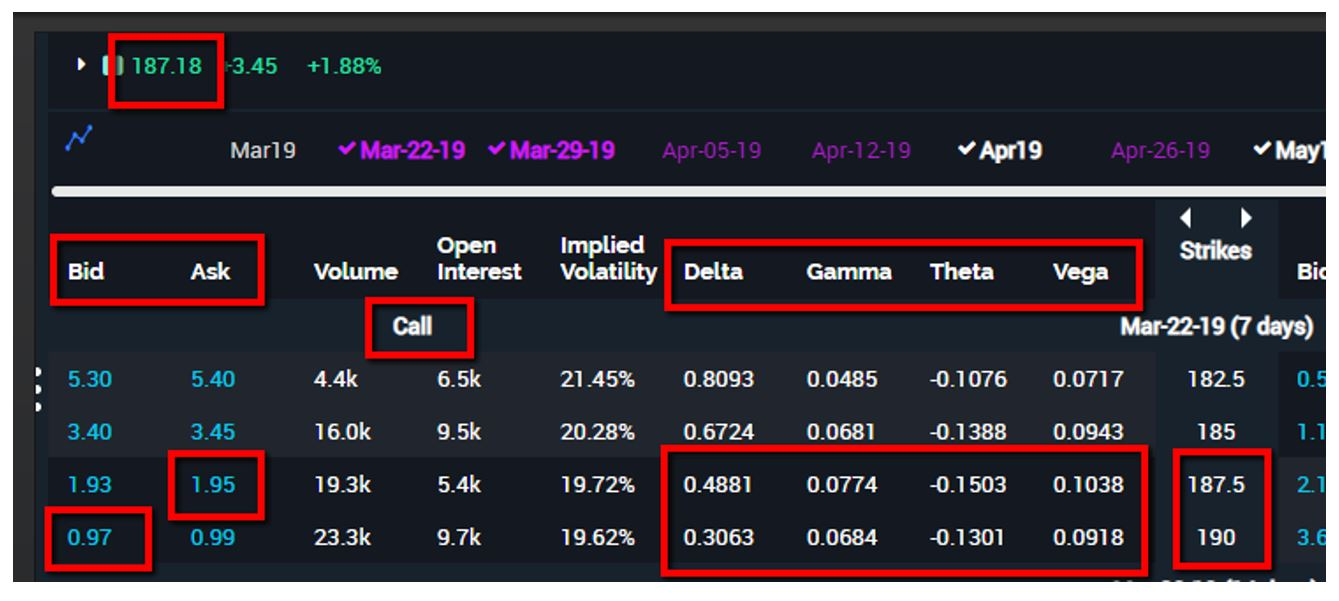If the 187.5/190 spread was chosen, the cost and maximum risk is 0.98 (1.95 – 0.97), which means the maximum profit is 1.52 (2.50 – 0.98) if the stock is trading at or above \$190 at expiration. The maximum loss is realized if the underlying closes at \$187.50 or lower at expiration. The breakeven is \$188.48 (187.50 + 0.98) at expiration. Delta is approximately positive 0.18 (0.4881 – 0.3063) and theta is approximately negative 0.02 (0.1301 – 0.1503).

But what if lower strikes were chosen? Take a look at the option chain below where the 185/187.5 bull call spread is chosen with the same expiration.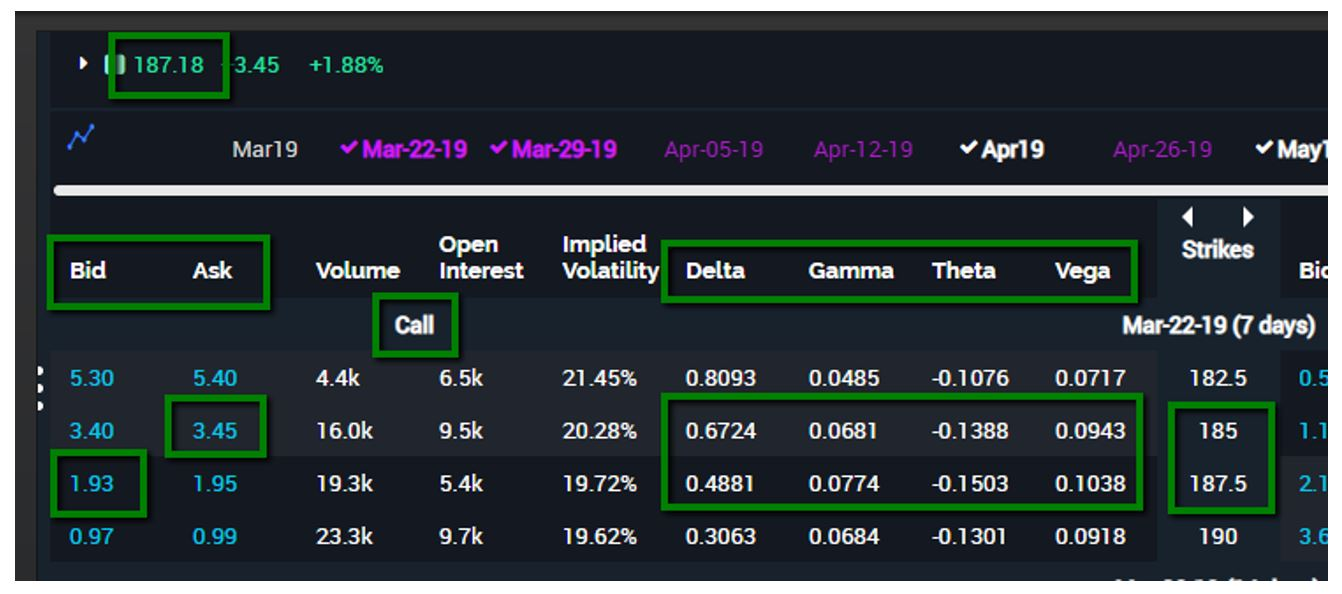The cost and maximum risk for this spread is 1.52 (3.45 – 1.93), which means the maximum profit is 0.98 (2.50 – 1.52) if the stock is trading at or above \$187.50 at expiration. The maximum loss is realized if the underlying closes at \$185 or lower at expiration. The breakeven is \$186.52 (185 + 1.52) at expiration. Delta is similar to the 187.5/190 spread. It is around positive 0.18 (0.6724 – 0.4881) and theta is close to positive 0.01 (0.1503 – 0.1388) instead of being negative like the 187.5/190 spread. Clearly the risk/reward ratio is better for the 187.5/190 (0.98/1.52) spread versus the 185/187.5 (1.52/0.98) spread. In fact, it is the opposite for this example. But does the risk/reward ratio tell the whole story?

Look at the maximum profit, maximum loss and breakevens. They are all lower for the 185/187.5 spread versus the 187.5/190 spread. An option trader may not want to risk everything and may not want to go for the maximum profit, but knowing there is more room for the underlying to move lower without potentially losing everything may help. In addition, the breakeven and maximum profit for the spread is closer for the 185/187.5 spread.

Getting back to the example, the stock moved higher but not as much as expected. Which spread did better? Take a look at the option chain below with the stock now trading at \$188.16 or up \$0.98 (188.16 – 187.18) from the purchase price.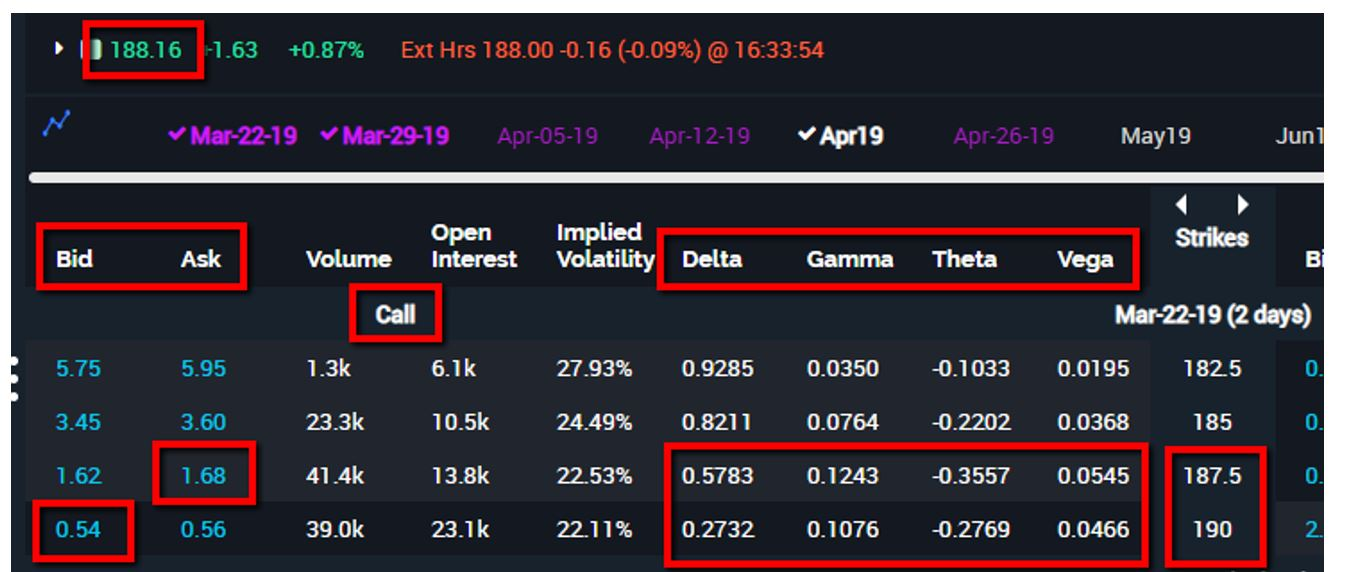The position is up \$0.16 ((1.68 – 0.54) – 0.98) based on the ask price of the spread. Not bad but now the negative theta has grown to approximately 0.08 (0.2769 – 0.3557) because the underlying is still trading below the breakeven and 5 days have passed with only 2 days left to expiration.

Taking a look at the option chain with the 185/187.5 spread, the spread is even more profitable and theta is even more positive than the original position as seen below.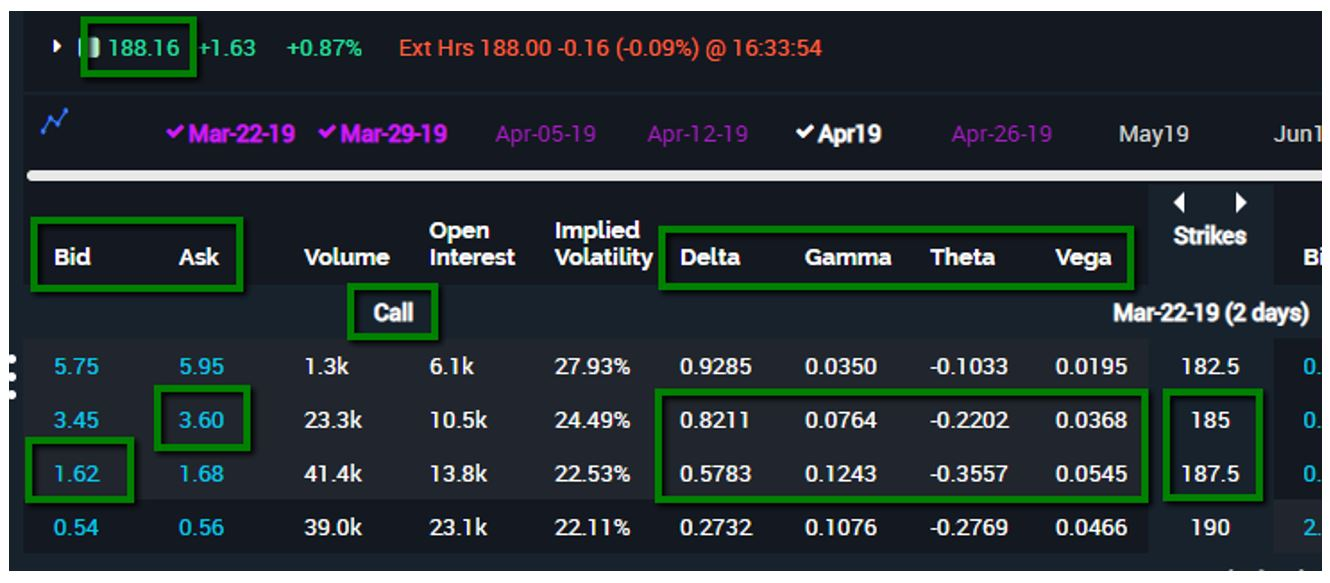The position is up \$0.46 ((3.60 – 1.62) – 1.52) based on the ask price of the spread. The positive theta has ballooned to approximately 0.14 (0.3557 – 0.2202) from about 0.01. Despite the greater risk/reward, the spread is more profitable and has a fair amount of positive theta on its side to help as time passes and the underlying does not move much lower. If 10 contracts were purchased, the profit would be \$300 ((0.46 – 0.16) X 10) greater for the 185/187.5 spread.

There are similarities between vertical debit and credit spreads. Many option traders do not take that into account when trading vertical debit spreads. A bigger initial delta and a move to either positive or more positive theta based on a shorter expiration and deeper ITM strikes should be debated when modeling the spreads. Many times, shorter and deeper is the better option so to speak, despite surrendering a larger risk/reward ratio.

John Kmiecik
Senior Options Instructor
Market Taker Mentoring, Inc.